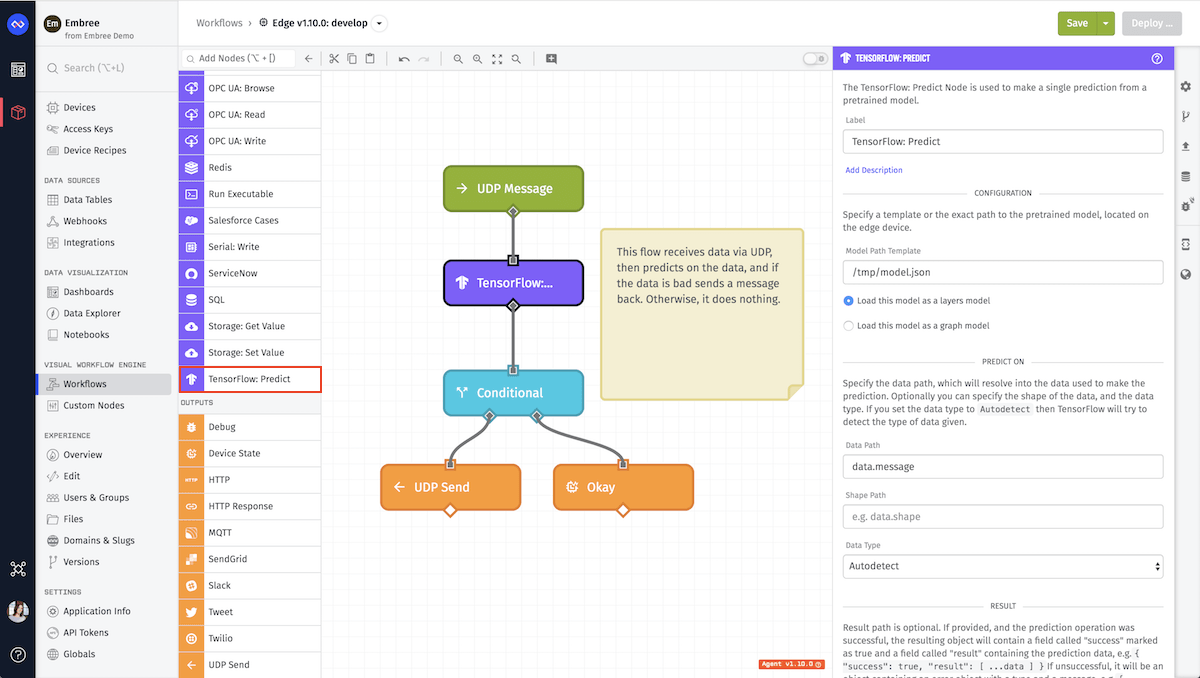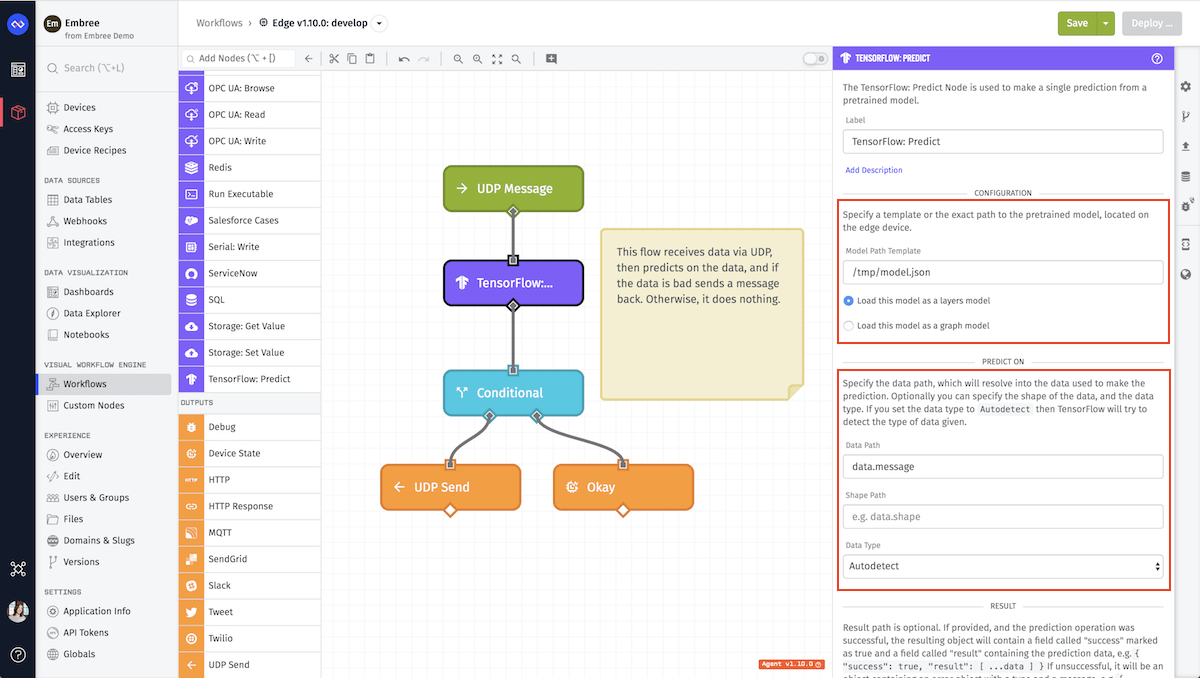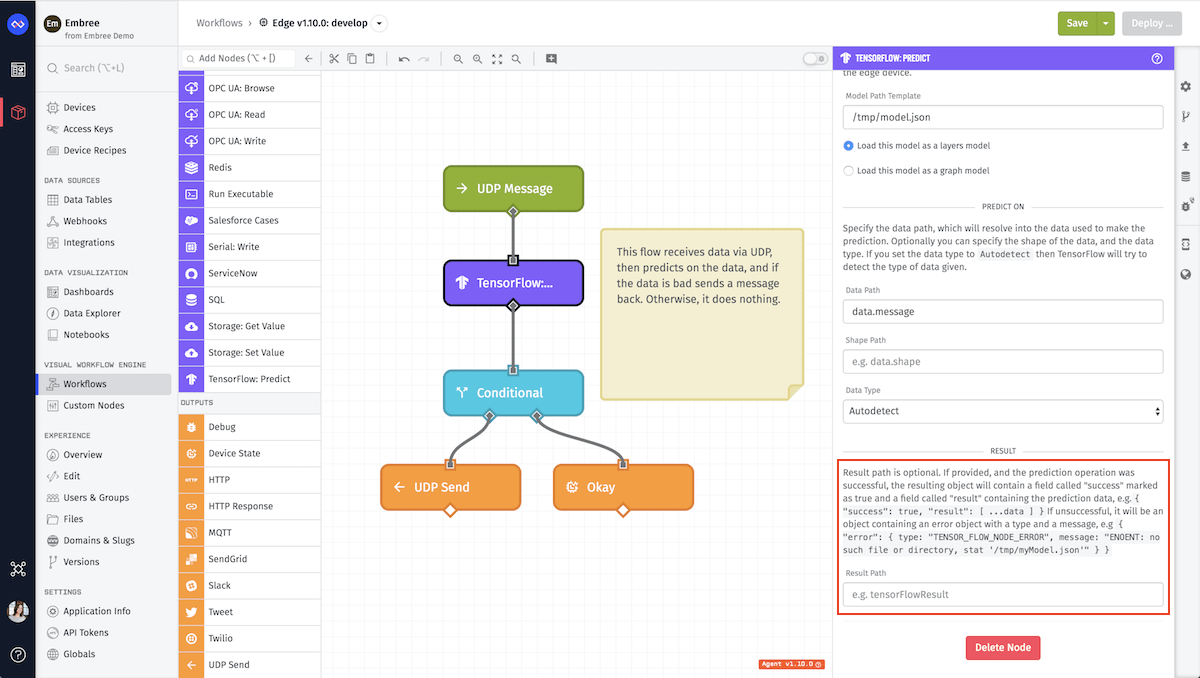Edge 1.10.0

# TensorFlow: Predict Node

The TensorFlow: Predict Node allow you to predict data on a pretrained TensorFlow model that has been loaded onto your edge device running Losant Edge Agent. The TensorFlow: Predict Node can load graph or layers model and predict on either of them.## Node Usage

This node can only load TensorFlow.js models. If you have a TensorFlow Python model, you can use a converter to turn it into the proper TensorFlow.js format.

This node is only available in Edge Workflows, and only for specific versions. The following architecture types of our Edge Agent support the TensorFlow: Predict Node:

• `arm32`
• `amd64`

The recommended way to make your model available to an Edge Workflow is to mount it using Docker Volumes. For example, you could mount it like so:

``````docker run -d --restart always --name docs-agent \
-v /path/to/your/model:/model \
-v /var/lib/losant-edge-agent/data:/data \
-v /var/lib/losant-edge-agent/config.toml:/etc/losant/losant-edge-agent-config.toml \
losant/edge-agent``````

If you replace `/path/to/your/model` with the correct path, your model will be available at `/model` within the Edge Agent Container.

## Node Properties

There are six pieces of configuration for this node.### Configuration

First, specify a template or the exact path to the pretrained model, located on the edge device.

• Model Path Template: (Required) This is the path to the model file that is on the edge device. This path should include the model file e.g. `/tmp/model.json`. The weights file should be put in the same directory, and will automatically be found by TensorFlow itself.
• Load Model as a layers model: (Default) By default this box is checked. This will interpret the model path as a layers model path using the tf.loadLayerModel method to load the model and it’s weights.bin file.
• Load Model as a graph model: If this is checked instead of the `Load Model as a layers model`, then the model path will be loaded as a graph model using the tf.loadGraphModel method.

### Predict On

Next, specify the data path, which will resolve into the data used to make the prediction.

• Date Path: (Required) The path to the nested array of data (tensor) to predict on.
• Shape Path: (Optional) The shape of the tensor (data path) given, if left blank it will be inferred by the array at the data path.
• Date Type: (Optional) This is the data type of the tensor, by default this is set to `Autodetect` which means it will be inferred by the tensor at the data path. The following at valid data types: `Autodetect`, `Float 32`, `Integer 32`, `Boolean`, `String`.

### Result Path

Result path is optional. If provided, and the prediction operation was successful, the resulting object will contain a field called `success` marked as true and a field called `result` containing the prediction data. If an error was returned from TensorFlow the result will be an object with an `error` field which will contain a `type` and `message` of the error.## Node Example

The following is an example of a successful prediction, where the user set the result path to `prediction`:

``````{
"prediction": {
"successful": true,
"result": [
0.928,
0.072,
0.928,
0.072
]
}
}``````

## Node Errors

This following is an example of a prediction failure if the path given was not found, where the user set the result path to `prediction`:

``````{
"prediction": {
"error": {
"type": "TENSOR_FLOW_NODE_ERROR",
"message": "ENOENT: no such file or directory, stat '/tmp/model.json'"
}
}
}``````